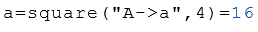# Class Square

Calculation of the properties of squares

## Description

The class Square calculates properties of a square.

The default argument is the side length $$a$$. This means that the side length can be passed as an argument without a command string (see example below).

## Properties

The following properties can be queried as a result or passed as an argument.

#### Description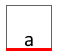a Length of $$a$$    (default)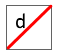d Diagonal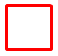P Perimeter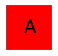A Area

## Syntax

Object = Square (a)

Object = Square ("arg names", arguments)

Value = Square ("get -> arg names", arguments)

## Example

Calculation of the properties with the side length $$a$$.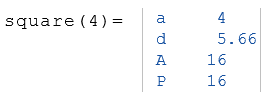Calculation of the properties with the diagonalen $$d$$.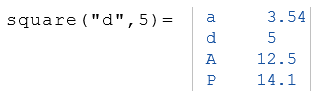The following example displays the area $$A$$ of the object $$a$$.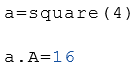Calculation of the area $$A$$ from the side length $$a$$.